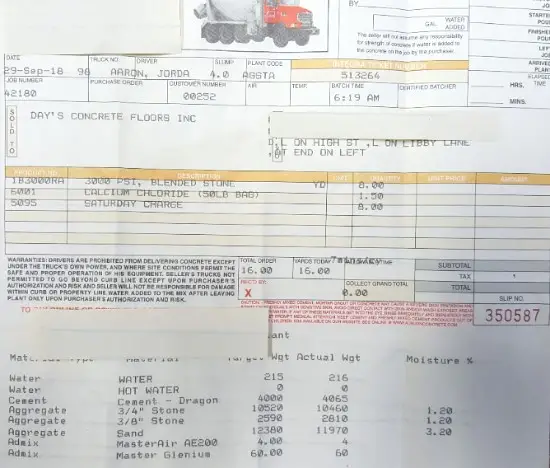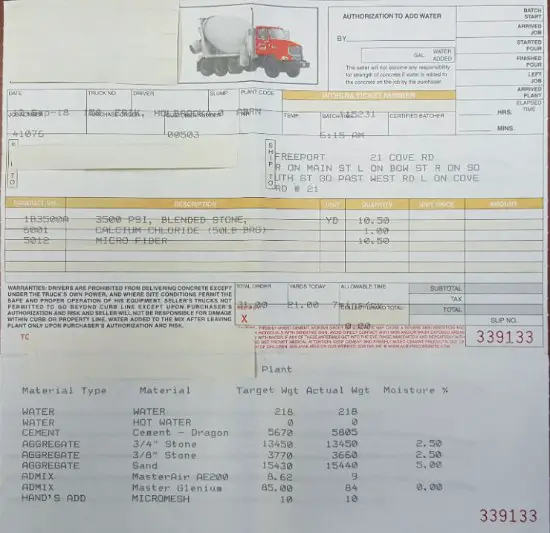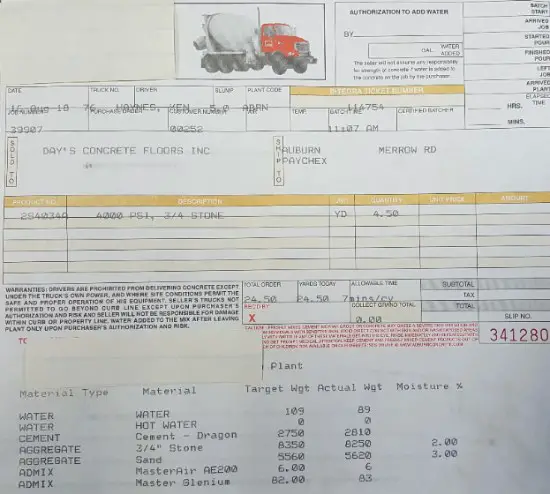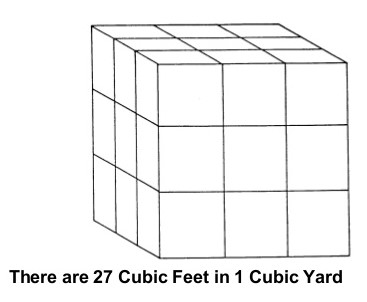# How much does a yard of concrete weigh?

Hi, Mike Day here, I own this website and I also own Day's Concrete Floors, Inc.

One of the ready mix concrete companies I buy my concrete from is Auburn Concrete Co.

According to Auburn Concrete Company, a yard of concrete weighs approximately 3700 pounds.

If you take a look below at the concrete tickets they give me after each load of concrete I use, they break down the weights for cement, sand, stone, and water.

The three different loads of concrete you see all average about 3700 pounds per cubic yard of concrete.The above ticket is for an 8 yard load of 3000 psi concrete. If you look at the Actual Weight of water, cement, aggregate (stone), and sand, the total weight for the 8 yards is 29,521 pounds.

If I divide the total pounds 29521 by total yards 8, I get 3,690 pounds per yard.

Take a look at another ticket below.The above ticket is for a 10.5 yard load of 3500 psi concrete.

The Actual weight for water, cement, aggregate (stone), and sand for all 10.5 yards is 38,573 pounds.

If I divide the total pounds 38573 by 10.5 yards, I get 3,674 pounds for each yard of concrete.

Take a look at one more ticket.This concrete ticket is for a 4.5 yard load of 4000 psi concrete.

The Actual weight for all water, cement, aggregate (stone), and sand is 16,769 pounds.

If I divide 16769 pounds by 4.5 yards I get 3,726 pounds per yard of concrete.

From my experience, most ready mix concrete companies will have a weight per cubic yard of concrete that's close to 3700 pounds.

Their weight per yard may fluctuate a little, but in general you can use 3700 pounds per cubic yard.

### How much does a yard of concrete weigh in tons?

In the U.S. a Ton is equal to 2000 pounds.

A yard of concrete weighs 3700 pounds.

If you divide 3700 pounds by 2000 pounds you get 1.85 Tons.

A cubic yard of concrete weighs 1.85 Tons.

If you use the metric system of measurement:

A cubic meter of concrete weighs 2400 kg.

A metric ton is equal to 1000 kg.

If you divide 2400kg by 1000kg you get 2.4 metric tons

A cubic meter of concrete weighs 2.4 metric tons.

### What is the weight of wet concrete vs dry concrete?

From the information above we know a yard of concrete weighs 3700 pounds. Most of the weight is from cement, stone and sand.

That weight isn't going to change whether the concrete is wet or dry.

If you look at the concrete tickets you'll see there's about 20 - 25 gallons of water used per yard of concrete.

1 gallon of water weighs about 8.34 pounds. This is what will change the weight of concrete when it goes from wet to dry.

When "wet" concrete dries or hardens, it uses about half to 2/3rds of this water in the process of hydration.

Some of the water evaporates as it cures and some of the water remains in the concrete for days, weeks, and even years.

Although the weight change is minimal, if 10 - 12 gallons of water is used up and/or evaporates, that's about 100 pounds per yard of concrete.

We can conclude:

A yard of wet concrete weighs 3700 pounds and a yard of dry concrete weighs about 3600 pounds.

Please don't take these numbers literal but just as a general reference.

### How much does a cubic foot of concrete weigh?A cubic foot of concrete is 12" wide by 12" high by 12" deep.

There are 27 cubic feet in a cubic yard of concrete.

From the concrete tickets above, a yard of concrete weighs 3700 pounds.

If you divide 3700 pounds by 27 you get 137 pounds per cubic foot.

A cubic foot of concrete weighs 137 pounds.

### How much does a square foot of concrete weigh?

A square foot is measured using length 12" by width 12".

If a cubic foot of concrete weighs 137 pounds.

The weight of a square foot of concrete will depend on how thick it is:

• 1 square foot x 8" thick concrete weighs 91.33 pounds
• 1 square foot x 6" thick concrete weighs 68.5 pounds
• 1 square foot x 4" thick concrete weighs 45.67 pounds
• 1 square foot x 2" thick concrete weighs 22.83 pounds

### How much does a yard of crushed concrete weigh?

The weight of crushed concrete will vary depending on the crush size.

According to Auburn Concrete, who also owns Auburn Aggregates:

• 1.5" crushed concrete weighs 2,460 pounds per yard
• 3/4" crushed concrete weighs 2,510 pounds per yard

Auburn Aggregates also sells crushed concrete by the ton:

• A ton of 1.5" crushed concrete costs \$7.00 per ton
• A ton of 3/4" crushed concrete costs \$8.00 per ton

### What is the weight of concrete per cubic meter?

A cubic meter of concrete weighs 2400 kg.

There are 1000 kg in a metric ton.

2400kg / 1000kg = 2.4 metric tons

A cubic meter of concrete also weighs 2.4 metric tons.

### What is the weight of concrete block?

There are a few different sizes of concrete blocks. I'll include some of the more popular sizes below.

• 8" x 12" x 16" concrete block weighs 40.5 pounds
• 8" x 8" x 16" concrete block weighs 36.5 pounds
• 8" x 8" x 8" concrete block weighs 18 pounds
• 4" x 8" x 16" solid concrete block weighs 33 pounds
• 4" x 8" x 16" regular concrete block weighs 24 pounds
• 6" x 8" x 16" concrete block weighs 25 pounds

Those are the most popular sizes of concrete blocks. I referenced Home Depot to get the weight of each block.

#### Check concrete weight using this concrete weight calculator

Inch calculator provides a concrete weight calculator so you can easily enter in your volume of concrete and find out how much the concrete weighs.

They are basing their calculations on a cubic yard of concrete weighing 4050 pounds, a cubic meter of concrete weighing 2400 kg, and a cubic foot of concrete weighing 150 lbs.

Slightly different than what the actual weights I show you above, but it'll give you a good idea of what concrete weighs.

Use it at your own discretion.